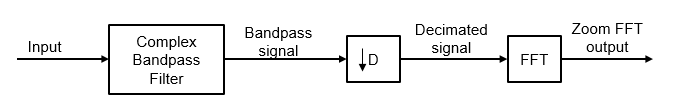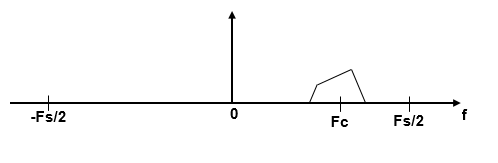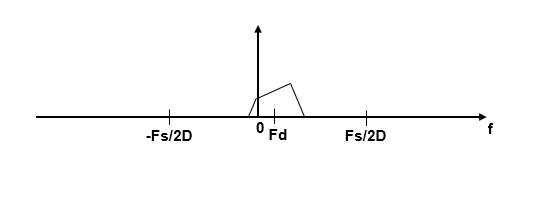Documentation

# dsp.ZoomFFT

High-resolution FFT of a portion of a spectrum

## Description

The `dsp.ZoomFFT` System object™ computes the fast Fourier Transform (FFT) of a signal over a portion of frequencies in the Nyquist interval. By setting an appropriate decimation factor D, and sampling rate Fs, you can choose the bandwidth of frequencies to analyze BW, where BW = Fs/D. You can also select a specific range of frequencies to analyze in the Nyquist interval by choosing the center frequency of the desired band.

The resolution of a signal is the ratio of Fs and the FFT length (L). Using zoom FFT, you can retain the same resolution you would achieve with a full-size FFT on your original signal by computing a small FFT on a shorter signal. The shorter signal comes from decimating the original signal. The savings come from being able to compute a much shorter FFT while achieving the same resolution. For a decimation factor of D, the new sampling rate, Fsd, is Fs/D, and the new frame size (and FFT length) is Ld = L/D. The resolution of the decimated signal is Fsd/Ld = Fs/L. To achieve a higher resolution of the shorter band, use the original FFT length, L, instead of the decimated FFT length, Ld.

To compute the FFT of a portion of the spectrum:

1. Create the `dsp.ZoomFFT` object and set its properties.

2. Call the object with arguments, as if it were a function.

## Creation

### Syntax

``zfft = dsp.ZoomFFT``
``zfft = dsp.ZoomFFT(d)``
``zfft = dsp.ZoomFFT(d,Fc)``
``zfft = dsp.ZoomFFT(d,Fc,Fs)``
``zfft = dsp.ZoomFFT(Name,Value)``

### Description

````zfft = dsp.ZoomFFT` creates a zoom FFT System object, `zfft`, that performs an FFT on a portion of the input signal's frequency range. The object determines the frequency range over which to perform the FFT using the specified center frequency and decimation factor values.```

example

````zfft = dsp.ZoomFFT(d)` creates a zoom FFT object with the DecimationFactor property set to `d`.```

example

````zfft = dsp.ZoomFFT(d,Fc)` creates a zoom FFT object with the DecimationFactor property set to `d`, and the CenterFrequency property set to `Fc`.```

example

````zfft = dsp.ZoomFFT(d,Fc,Fs)` creates a zoom FFT object with the DecimationFactor property set to `d`, the CenterFrequency property set to `Fc`, and the SampleRate property set to `Fs`.```

example

````zfft = dsp.ZoomFFT(Name,Value)` creates a zoom FFT object with each specified property set to the specified value. Enclose each property name in single quotes. You can use this syntax with any previous input argument combinations.Example: ```zfft = dsp.ZoomFFT(2,2e3,48e3,'FFTLength',64);``````

## Properties

expand all

Unless otherwise indicated, properties are nontunable, which means you cannot change their values after calling the object. Objects lock when you call them, and the `release` function unlocks them.

If a property is tunable, you can change its value at any time.

Decimation factor, specified as a positive integer. This value specifies the factor by which the object reduces the bandwidth of the input signal. The number of rows in the input signal must be a multiple of the decimation factor.

Example: `4`

Example: `8`

Data Types: `single` | `double` | `int8` | `int16` | `int32` | `int64` | `uint8` | `uint16` | `uint32` | `uint64`

Center frequency of the desired band in Hz, specified as a real scalar in the range (– `SampleRate`/2, `SampleRate`/2).

Example: `0.5`

Example: `10`

Tunable: Yes

Data Types: `single` | `double`

FFT length, specified as a positive integer. The FFT length must be greater than or equal to the ratio of the frame size (number of input rows) and the decimation factor, L/D. The default, `[]`, specifies an FFT length that equals the ratio, L/D.

Example: `24`

Example: `52`

Data Types: `single` | `double` | `int8` | `int16` | `int32` | `int64` | `uint8` | `uint16` | `uint32` | `uint64`

Input sample rate in Hz, specified as positive real scalar.

Example: `44100`

Example: `48000`

Data Types: `single` | `double`

## Usage

### Syntax

``zfftOut = zfft(input)``

### Description

example

````zfftOut = zfft(input)` computes the zoom FFT of the input. Each column of the input is treated as an independent channel. The object computes the FFT of each channel of the input signal independently over time.```

### Input Arguments

expand all

Data input whose zoom FFT the object computes, specified as a vector or a matrix. The number of input rows must be a multiple of the decimation factor.

This object supports variable-size input signals, as long as the input frame size is a multiple of the decimation factor. That is, you can change the input frame size (number of rows) even after calling the algorithm. However, the number of channels (number of columns) must remain constant.

Example: `randn(22,2)`

Data Types: `single` | `double`
Complex Number Support: Yes

### Output Arguments

expand all

Zoom FFT output, returned as a vector or matrix. If the FFT length is set to auto, the output frame size equals the input frame size divided by the decimation factor. If the object specifies the FFT length, the output frame size equals the specified FFT length. The output data type matches the input data type.

Example: randn(11,2)

Data Types: `single` | `double`
Complex Number Support: Yes

## Object Functions

To use an object function, specify the System object as the first input argument. For example, to release system resources of a System object named `obj`, use this syntax:

`release(obj)`

expand all

 `step` Run System object algorithm `release` Release resources and allow changes to System object property values and input characteristics `reset` Reset internal states of System object

## Examples

expand all

Compute FFT of the [1500 Hz 2500 Hz] subband using zoom FFT for a signal sampled at 48 kHz.

Initialization

Set the center frequency to 2 kHz and the bandwidth of interest to 1 kHz. The bandwidth is centered at the center frequency. The decimation factor is the ratio of the input sample rate, 48 kHz, and the bandwidth of interest, 1 kHz. Choose an FFT length of 64. Set the input frame size to be the decimation factor times the FFT length. Create a `dsp.ZoomFFT` object with the specified decimation factor, center frequency, sample rate, and FFT length.

```Fs = 48e3; CF = 2e3; BW = 1e3; D = Fs/BW; fftlen = 64; L = D * fftlen; zfft = dsp.ZoomFFT(D,CF,Fs,'FFTLength',fftlen); ```

Frequencies

The FFT is computed over frequencies starting at 1500 Hz and spaced by \$Fs/(D*fftLen)\$Hz apart, which is the resolution or the minimum frequency that can be discriminated. The number of frequencies at which the zoom FFT is computed equals the FFT length.

```Fsd = Fs/D; F = CF + (Fsd/fftlen)*(0:fftlen-1)-Fsd/2; ```

Initialize the Scope

Create an array plot to show the frequencies in `F`.

```ap = dsp.ArrayPlot('XDataMode','Custom','CustomXData',F,... 'YLabel','z .* conj(z)','XLabel','Frequency (Hz)','YLimits',[0 1.1e3],... 'Title',sprintf('Decimation Factor = %d. Center Frequency = %d Hz. Resolution = %f Hz',D, CF,(Fs/D)/fftlen)); ```

Sine Wave Generator

Create a sine wave with frequencies at 1625 Hz, 2000 Hz, and 2125 Hz.

```tones = [1625 2000 2125]; sine = dsp.SineWave('SampleRate',Fs,'Frequency',tones,'SamplesPerFrame',L); ```

Streaming

Pass a noisy sine wave with a sample rate of 48 kHz. Compute the zoom FFT of this sine wave in the subband [1500 Hz 2500 Hz]. Rearrange the Fourier transform by shifting the zero-frequency component to the center of the array. View the tones at 1625 Hz, 2000 Hz, and 2125 Hz in the array plot.

```for i = 1:1000 x = sum(sine(),2)+1e-1*randn(L,1); z = zfft(x); z = fftshift(z); ap(z.*conj(z)); end ```The `dsp.ZoomFFT` object accepts variable-size inputs as long as the input is a multiple of the decimation factor. The number of input channels cannot change.

Create a `dsp.ZoomFFT` object with a decimation factor of 4, center frequency of 2 kHz, and an input sample rate of 48 kHz. Pass a random input with 4*64 rows and 2 columns. Vary the number of rows to 4*128 and 4*32. The resulting FFT lengths are 64, 128, and 32, respectively. The size of the outputs is [64 2], [128 2], and [32 2], respectively.

```zfft = dsp.ZoomFFT(4,2e3,48e3); y1 = zfft(randn(4*64,2)); y2 = zfft(randn(4*128,2)); y3 = zfft(randn(4*32,2));```

Set the FFT length as 256 and pass variable-size inputs. The size of all the outputs is [256 2].

```release(zfft); zfft.FFTLength = 256; y4 = zfft(randn(4*64,2)); y5 = zfft(randn(4*128,2)); y6 = zfft(randn(4*32,2));```

## Algorithms

The zoom FFT algorithm leverages bandpass filtering before computing the FFT of the signal. The concept of bandpass filtering is that suppose you are interested in the band [F1, F2] of the original input signal, sampled at the rate Fs Hz. If you pass this signal through a complex (one-sided) bandpass filter centered at Fc = (F1+F2)/2, with the bandwidth BW = F2F1, and then downsample the signal by a factor of D = floor(Fs/BW), the desired band comes down to the baseband.If Fc cannot be expressed in the form of k×Fs/D, where k is an integer, then the shifted, decimated spectrum is not centered at DC. In this case, the center frequency gets translated to Fd.

`${F}_{d}={F}_{c}-\left({F}_{s}/D\right)×floor\left(\left(D×{F}_{c}+{F}_{s}/2\right)/{F}_{s}\right)$`

The complex bandpass filter is obtained by first designing a lowpass filter prototype and then multiplying the lowpass coefficients with a complex exponential. This algorithm uses a multirate, multistage FIR filter as the lowpass filter prototype. To obtain the bandpass filter, the coefficients of each stage are frequency shifted. The decimation factor is the cumulative decimation factor of each stage. The complex bandpass filter followed by the decimator are implemented using an efficient polyphase structure. For more details on the design of the complex bandpass filter from the multirate multistage FIR filter prototype, see Zoom FFT and Complex Bandpass Filter Design.

 Harris, F.J. Multirate Signal Processing for Communication Systems. Prentice Hall, 2004, pp. 208–209.

Watch now